# 图像处理边缘检测点检测

## 图像处理常用边缘检测算子总结

2013-06-05 10:20:42 Augusdi 阅读数 96257
• ###### matlab边缘检测和图像分割

matlab边缘检测 <img src="https://img-bss.csdn.net/201903190616489027.jpg" alt="" /></p>

7课时 101分钟 981人学习 刘昱显
免费试看

不同图像灰度不同，边界处一般会有明显的边缘，利用此特征可以分割图像。需要说明的是：缘和物体间的边界并不等同，边缘指的是图像中像素的值有突变的地方，而物体间的边界指的是现实场景中的存在于物体之间的边界。有可能有边缘的地方并非边界，也有可能边界的地方并无边缘，因为现实世界中的物体是三维的，而图像只具有二维信息，从三维到二维的投影成像不可避免的会丢失一部分信息；另外，成像过程中的光照和噪声也是不可避免的重要因素。正是因为这些原因，基于边缘的图像分割仍然是当前图像研究中的世界级难题，目前研究者正在试图在边缘提取中加入高层的语义信息。

在实际的图像分割中，往往只用到一阶和二阶导数，虽然，原理上，可以用更高阶的导数，但是，因为噪声的影响，在纯粹二阶的导数操作中就会出现对噪声的敏感现象，三阶以上的导数信息往往失去了应用价值。二阶导数还可以说明灰度突变的类型。在有些情况下，如灰度变化均匀的图像，只利用一阶导数可能找不到边界，此时二阶导数就能提供很有用的信息。二阶导数对噪声也比较敏感，解决的方法是先对图像进行平滑滤波，消除部分噪声，再进行边缘检测。不过，利用二阶导数信息的算法是基于过零检测的，因此得到的边缘点数比较少，有利于后继的处理和识别工作。

各种算子的存在就是对这种导数分割原理进行的实例化计算，是为了在计算过程中直接使用的一种计算单位。

1.Sobel算子

其主要用于边缘检测，在技术上它是以离散型的差分算子，用来运算图像亮度函数的梯度的近似值， Sobel算子是典型的基于一阶导数的边缘检测算子，由于该算子中引入了类似局部平均的运算，因此对噪声具有平滑作用，能很好的消除噪声的影响。Sobel算子对于象素的位置的影响做了加权，与Prewitt算子、Roberts算子相比因此效果更好。

Sobel算子包含两组3x3的矩阵，分别为横向及纵向模板，将之与图像作平面卷积，即可分别得出横向及纵向的亮度差分近似值。实际使用中，常用如下两个模板来检测图像边缘。

2. Isotropic Sobel算子

Sobel算子另一种形式是(Isotropic Sobel)算子，加权平均算子，权值反比于邻点与中心点的距离，当沿不同方向检测边缘时梯度幅度一致，就是通常所说的各向同性Sobel(Isotropic Sobel)算子。模板也有两个，一个是检测水平边沿的 ，另一个是检测垂直平边沿的 。各向同性Sobel算子和普通Sobel算子相比，它的位置加权系数更为准确，在检测不同方向的边沿时梯度的幅度一致。

3. Roberts算子

Roberts边缘算子是一个2x2的模板，采用的是对角方向相邻的两个像素之差。从图像处理的实际效果来看，边缘定位较准，对噪声敏感。适用于边缘明显且噪声较少的图像分割。Roberts边缘检测算子是一种利用局部差分算子寻找边缘的算子,Robert算子图像处理后结果边缘不是很平滑。经分析，由于Robert算子通常会在图像边缘附近的区域内产生较宽的响应，故采用上述算子检测的边缘图像常需做细化处理，边缘定位的精度不是很高。

4. Prewitt算子

Prewitt算子是一种一阶微分算子的边缘检测，利用像素点上下、左右邻点的灰度差，在边缘处达到极值检测边缘，去掉部分伪边缘，对噪声具有平滑作用 。其原理是在图像空间利用两个方向模板与图像进行邻域卷积来完成的，这两个方向模板一个检测水平边缘，一个检测垂直边缘。

对数字图像f(x，y)，Prewitt算子的定义如下：

G(i)=|[f(i-1,j-1)+f(i-1,j)+f(i-1，j+1)]-[f(i+1,j-1)+f(i+1，j)+f(i+1，j+1)]|
G(j)=|[f(i-1,j+1)+f(i,j+1)+f(i+1，j+1)]-[f(i-1,j-1)+f(i,j-1)+f(i+1，j-1)]|

Prewitt算子对噪声有抑制作用，抑制噪声的原理是通过像素平均，但是像素平均相当于对图像的低通滤波，所以Prewitt算子对边缘的定位不如Roberts算子。

5.Laplacian算子

Laplace算子是一种各向同性算子，二阶微分算子，在只关心边缘的位置而不考虑其周围的象素灰度差值时比较合适。Laplace算子对孤立象素的响应要比对边缘或线的响应要更强烈，因此只适用于无噪声图象。存在噪声情况下，使用Laplacian算子检测边缘之前需要先进行低通滤波。所以，通常的分割算法都是把Laplacian算子和平滑算子结合起来生成一个新的模板。

拉式算子用来改善因扩散效应的模糊特别有效，因为它符合降制模型。扩散效应是成像过程中经常发生的现象。

Laplacian算子一般不以其原始形式用于边缘检测，因为其作为一个二阶导数，Laplacian算子对噪声具有无法接受的敏感性；同时其幅值产生算边缘，这是复杂的分割不希望有的结果；最后Laplacian算子不能检测边缘的方向；所以Laplacian在分割中所起的作用包括：（1）利用它的零交叉性质进行边缘定位；（2）确定一个像素是在一条边缘暗的一面还是亮的一面；一般使用的是高斯型拉普拉斯算子（Laplacian of a Gaussian,LoG)，由于二阶导数是线性运算，利用LoG卷积一幅图像与首先使用高斯型平滑函数卷积改图像，然后计算所得结果的拉普拉斯是一样的。所以在LoG公式中使用高斯函数的目的就是对图像进行平滑处理，使用Laplacian算子的目的是提供一幅用零交叉确定边缘位置的图像；图像的平滑处理减少了噪声的影响并且它的主要作用还是抵消由Laplacian算子的二阶导数引起的逐渐增加的噪声影响。

6.Canny算子

该算子功能比前面几种都要好，但是它实现起来较为麻烦，Canny算子是一个具有滤波，增强，检测的多阶段的优化算子，在进行处理前，Canny算子先利用高斯平滑滤波器来平滑图像以除去噪声，Canny分割算法采用一阶偏导的有限差分来计算梯度幅值和方向，在处理过程中，Canny算子还将经过一个非极大值抑制的过程，最后Canny算子还采用两个阈值来连接边缘。

Canny边缘检测算法

step1: 用高斯滤波器平滑图象；

step2: 用一阶偏导的有限差分来计算梯度的幅值和方向；

step3: 对梯度幅值进行非极大值抑制

step4: 用双阈值算法检测和连接边缘

(1)图象边缘检测必须满足两个条件：一能有效地抑制噪声；二必须尽量精确确定边缘的位置。

(2)根据对信噪比与定位乘积进行测度，得到最优化逼近算子。这就是Canny边缘检测算子。

(3)类似与Marr（LoG）边缘检测方法，也属于先平滑后求导数的方法。

## 图像处理之Canny边缘检测

2014-11-16 16:50:47 jia20003 阅读数 62627
• ###### matlab边缘检测和图像分割

matlab边缘检测 <img src="https://img-bss.csdn.net/201903190616489027.jpg" alt="" /></p>

7课时 101分钟 981人学习 刘昱显
免费试看

Canny边缘检测算法是1986年有John F. Canny开发出来一种基于图像梯度计算的边缘

。但是在实际工程应用中，考虑到输入图像都是彩色图像，最终边缘连接之后的图像要

1.      彩色图像转换为灰度图像

2.      对图像进行高斯模糊

3.      计算图像梯度，根据梯度计算图像边缘幅值与角度

4.      非最大信号压制处理（边缘细化）

5.      双阈值边缘连接处理

6.      二值化图像输出结果

1.      彩色图像转灰度图像

int gray = (int) (0.299 * tr + 0.587 * tg + 0.114 * tb);

2.      对图像进行高斯模糊

		float kernel[][] = new float[gaussianKernelWidth][gaussianKernelWidth];
for(int x=0; x<gaussianKernelWidth; x++)
{
for(int y=0; y<gaussianKernelWidth; y++)
{
}
}

// 高斯模糊 -灰度图像
for (int row = 0; row < height; row++) {
for (int col = 0; col < width; col++) {
index = row * width + col;
double weightSum = 0.0;
double redSum = 0;
for(int subRow=-krr; subRow<=krr; subRow++)
{
int nrow = row + subRow;
if(nrow >= height || nrow < 0)
{
nrow = 0;
}
for(int subCol=-krr; subCol<=krr; subCol++)
{
int ncol = col + subCol;
if(ncol >= width || ncol <=0)
{
ncol = 0;
}
int index2 = nrow * width + ncol;
int tr1 = (inPixels[index2] >> 16) & 0xff;
redSum += tr1*kernel[subRow+krr][subCol+krr];
weightSum += kernel[subRow+krr][subCol+krr];
}
}
int gray = (int)(redSum / weightSum);
outPixels[index] = gray;
}
}

3.      计算图像X方向与Y方向梯度，根据梯度计算图像边缘幅值与角度大小

http://blog.csdn.net/jia20003/article/details/7664777。// 计算梯度-gradient, X放与Y方向
data = new float[width * height];
magnitudes = new float[width * height];
for (int row = 0; row < height; row++) {
for (int col = 0; col < width; col++) {
index = row * width + col;
// 计算X方向梯度
float xg = (getPixel(outPixels, width, height, col, row+1) -
getPixel(outPixels, width, height, col, row) +
getPixel(outPixels, width, height, col+1, row+1) -
getPixel(outPixels, width, height, col+1, row))/2.0f;
float yg = (getPixel(outPixels, width, height, col, row)-
getPixel(outPixels, width, height, col+1, row) +
getPixel(outPixels, width, height, col, row+1) -
getPixel(outPixels, width, height, col+1, row+1))/2.0f;
// 计算振幅与角度
data[index] = hypot(xg, yg);
if(xg == 0)
{
if(yg > 0)
{
magnitudes[index]=90;
}
if(yg < 0)
{
magnitudes[index]=-90;
}
}
else if(yg == 0)
{
magnitudes[index]=0;
}
else
{
magnitudes[index] = (float)((Math.atan(yg/xg) * 180)/Math.PI);
}
// make it 0 ~ 180
magnitudes[index] += 90;
}
}

4.      非最大信号压制// 非最大信号压制算法 3x3
Arrays.fill(magnitudes, 0);
for (int row = 0; row < height; row++) {
for (int col = 0; col < width; col++) {
index = row * width + col;
float angle = magnitudes[index];
float m0 = data[index];
magnitudes[index] = m0;
if(angle >=0 && angle < 22.5) // angle 0
{
float m1 = getPixel(data, width, height, col-1, row);
float m2 = getPixel(data, width, height, col+1, row);
if(m0 < m1 || m0 < m2)
{
magnitudes[index] = 0;
}
}
else if(angle >= 22.5 && angle < 67.5) // angle +45
{
float m1 = getPixel(data, width, height, col+1, row-1);
float m2 = getPixel(data, width, height, col-1, row+1);
if(m0 < m1 || m0 < m2)
{
magnitudes[index] = 0;
}
}
else if(angle >= 67.5 && angle < 112.5) // angle 90
{
float m1 = getPixel(data, width, height, col, row+1);
float m2 = getPixel(data, width, height, col, row-1);
if(m0 < m1 || m0 < m2)
{
magnitudes[index] = 0;
}
}
else if(angle >=112.5 && angle < 157.5) // angle 135 / -45
{
float m1 = getPixel(data, width, height, col-1, row-1);
float m2 = getPixel(data, width, height, col+1, row+1);
if(m0 < m1 || m0 < m2)
{
magnitudes[index] = 0;
}
}
else if(angle >=157.5) // 跟零度是一致的，感谢一位网友发现了这个问题
{
float m1 = getPixel(data, width, height, col+1, row);
float m2 = getPixel(data, width, height, col-1, row);
if(m0 < m1 || m0 < m2)
{
magnitudes[index] = 0;
}
}
}
}

1.      双阈值边缘连接

a.      对于任意边缘像素低于TL的则丢弃

b.      对于任意边缘像素高于TH的则保留

c.      对于任意边缘像素值在TL与TH之间的，如果能通过边缘连接到一个像素大于

TH而且边缘所有像素大于最小阈值TL的则保留，否则丢弃。代码实现如下：

Arrays.fill(data, 0);
int offset = 0;
for (int row = 0; row < height; row++) {
for (int col = 0; col < width; col++) {
if(magnitudes[offset] >= highThreshold && data[offset] == 0)
{
}
offset++;
}
}

private void edgeLink(int x1, int y1, int index, float threshold) {
int x0 = (x1 == 0) ? x1 : x1 - 1;
int x2 = (x1 == width - 1) ? x1 : x1 + 1;
int y0 = y1 == 0 ? y1 : y1 - 1;
int y2 = y1 == height -1 ? y1 : y1 + 1;

data[index] = magnitudes[index];
for (int x = x0; x <= x2; x++) {
for (int y = y0; y <= y2; y++) {
int i2 = x + y * width;
if ((y != y1 || x != x1)
&& data[i2] == 0
&& magnitudes[i2] >= threshold) {
return;
}
}
}
}

6.      结果二值化显示 - 不说啦，直接点，自己看吧，太简单啦

// 二值化显示
for(int i=0; i<inPixels.length; i++)
{
int gray = clamp((int)data[i]);
outPixels[i] = gray > 0 ? -1 : 0xff000000;
}package com.gloomyfish.filter.study;

import java.awt.image.BufferedImage;
import java.util.Arrays;

public class CannyEdgeFilter extends AbstractBufferedImageOp {
private int gaussianKernelWidth = 16;
private float lowThreshold;
private float highThreshold;
// image width, height
private int width;
private int height;
private float[] data;
private float[] magnitudes;

public CannyEdgeFilter() {
lowThreshold = 2.5f;
highThreshold = 7.5f;
gaussianKernelWidth = 16;
}

}

}

public int getGaussianKernelWidth() {
return gaussianKernelWidth;
}

public void setGaussianKernelWidth(int gaussianKernelWidth) {
this.gaussianKernelWidth = gaussianKernelWidth;
}

public float getLowThreshold() {
return lowThreshold;
}

public void setLowThreshold(float lowThreshold) {
this.lowThreshold = lowThreshold;
}

public float getHighThreshold() {
return highThreshold;
}

public void setHighThreshold(float highThreshold) {
this.highThreshold = highThreshold;
}

@Override
public BufferedImage filter(BufferedImage src, BufferedImage dest) {
width = src.getWidth();
height = src.getHeight();
if (dest == null)
dest = createCompatibleDestImage(src, null);
// 图像灰度化
int[] inPixels = new int[width * height];
int[] outPixels = new int[width * height];
getRGB(src, 0, 0, width, height, inPixels);
int index = 0;
for (int row = 0; row < height; row++) {
int ta = 0, tr = 0, tg = 0, tb = 0;
for (int col = 0; col < width; col++) {
index = row * width + col;
ta = (inPixels[index] >> 24) & 0xff;
tr = (inPixels[index] >> 16) & 0xff;
tg = (inPixels[index] >> 8) & 0xff;
tb = inPixels[index] & 0xff;
int gray = (int) (0.299 * tr + 0.587 * tg + 0.114 * tb);
inPixels[index] = (ta << 24) | (gray << 16) | (gray << 8)
| gray;
}
}

// 计算高斯卷积核
float kernel[][] = new float[gaussianKernelWidth][gaussianKernelWidth];
for(int x=0; x<gaussianKernelWidth; x++)
{
for(int y=0; y<gaussianKernelWidth; y++)
{
}
}
// 高斯模糊 -灰度图像
for (int row = 0; row < height; row++) {
for (int col = 0; col < width; col++) {
index = row * width + col;
double weightSum = 0.0;
double redSum = 0;
for(int subRow=-krr; subRow<=krr; subRow++)
{
int nrow = row + subRow;
if(nrow >= height || nrow < 0)
{
nrow = 0;
}
for(int subCol=-krr; subCol<=krr; subCol++)
{
int ncol = col + subCol;
if(ncol >= width || ncol <=0)
{
ncol = 0;
}
int index2 = nrow * width + ncol;
int tr1 = (inPixels[index2] >> 16) & 0xff;
redSum += tr1*kernel[subRow+krr][subCol+krr];
weightSum += kernel[subRow+krr][subCol+krr];
}
}
int gray = (int)(redSum / weightSum);
outPixels[index] = gray;
}
}

data = new float[width * height];
magnitudes = new float[width * height];
for (int row = 0; row < height; row++) {
for (int col = 0; col < width; col++) {
index = row * width + col;
// 计算X方向梯度
float xg = (getPixel(outPixels, width, height, col, row+1) -
getPixel(outPixels, width, height, col, row) +
getPixel(outPixels, width, height, col+1, row+1) -
getPixel(outPixels, width, height, col+1, row))/2.0f;
float yg = (getPixel(outPixels, width, height, col, row)-
getPixel(outPixels, width, height, col+1, row) +
getPixel(outPixels, width, height, col, row+1) -
getPixel(outPixels, width, height, col+1, row+1))/2.0f;
// 计算振幅与角度
data[index] = hypot(xg, yg);
if(xg == 0)
{
if(yg > 0)
{
magnitudes[index]=90;
}
if(yg < 0)
{
magnitudes[index]=-90;
}
}
else if(yg == 0)
{
magnitudes[index]=0;
}
else
{
magnitudes[index] = (float)((Math.atan(yg/xg) * 180)/Math.PI);
}
// make it 0 ~ 180
magnitudes[index] += 90;
}
}

// 非最大信号压制算法 3x3
Arrays.fill(magnitudes, 0);
for (int row = 0; row < height; row++) {
for (int col = 0; col < width; col++) {
index = row * width + col;
float angle = magnitudes[index];
float m0 = data[index];
magnitudes[index] = m0;
if(angle >=0 && angle < 22.5) // angle 0
{
float m1 = getPixel(data, width, height, col-1, row);
float m2 = getPixel(data, width, height, col+1, row);
if(m0 < m1 || m0 < m2)
{
magnitudes[index] = 0;
}
}
else if(angle >= 22.5 && angle < 67.5) // angle +45
{
float m1 = getPixel(data, width, height, col+1, row-1);
float m2 = getPixel(data, width, height, col-1, row+1);
if(m0 < m1 || m0 < m2)
{
magnitudes[index] = 0;
}
}
else if(angle >= 67.5 && angle < 112.5) // angle 90
{
float m1 = getPixel(data, width, height, col, row+1);
float m2 = getPixel(data, width, height, col, row-1);
if(m0 < m1 || m0 < m2)
{
magnitudes[index] = 0;
}
}
else if(angle >=112.5 && angle < 157.5) // angle 135 / -45
{
float m1 = getPixel(data, width, height, col-1, row-1);
float m2 = getPixel(data, width, height, col+1, row+1);
if(m0 < m1 || m0 < m2)
{
magnitudes[index] = 0;
}
}
else if(angle >=157.5) // angle 0
{
float m1 = getPixel(data, width, height, col, row+1);
float m2 = getPixel(data, width, height, col, row-1);
if(m0 < m1 || m0 < m2)
{
magnitudes[index] = 0;
}
}
}
}
// 寻找最大与最小值
float min = 255;
float max = 0;
for(int i=0; i<magnitudes.length; i++)
{
if(magnitudes[i] == 0) continue;
min = Math.min(min, magnitudes[i]);
max = Math.max(max, magnitudes[i]);
}
System.out.println("Image Max Gradient = " + max + " Mix Gradient = " + min);

// 通常比值为 TL : TH = 1 : 3， 根据两个阈值完成二值化边缘连接
Arrays.fill(data, 0);
int offset = 0;
for (int row = 0; row < height; row++) {
for (int col = 0; col < width; col++) {
if(magnitudes[offset] >= highThreshold && data[offset] == 0)
{
}
offset++;
}
}

// 二值化显示
for(int i=0; i<inPixels.length; i++)
{
int gray = clamp((int)data[i]);
outPixels[i] = gray > 0 ? -1 : 0xff000000;
}
setRGB(dest, 0, 0, width, height, outPixels );
return dest;
}

public int clamp(int value) {
return value > 255 ? 255 :
(value < 0 ? 0 : value);
}

private void edgeLink(int x1, int y1, int index, float threshold) {
int x0 = (x1 == 0) ? x1 : x1 - 1;
int x2 = (x1 == width - 1) ? x1 : x1 + 1;
int y0 = y1 == 0 ? y1 : y1 - 1;
int y2 = y1 == height -1 ? y1 : y1 + 1;

data[index] = magnitudes[index];
for (int x = x0; x <= x2; x++) {
for (int y = y0; y <= y2; y++) {
int i2 = x + y * width;
if ((y != y1 || x != x1)
&& data[i2] == 0
&& magnitudes[i2] >= threshold) {
return;
}
}
}
}

private float getPixel(float[] input, int width, int height, int col,
int row) {
if(col < 0 || col >= width)
col = 0;
if(row < 0 || row >= height)
row = 0;
int index = row * width + col;
return input[index];
}

private float hypot(float x, float y) {
return (float) Math.hypot(x, y);
}

private int getPixel(int[] inPixels, int width, int height, int col,
int row) {
if(col < 0 || col >= width)
col = 0;
if(row < 0 || row >= height)
row = 0;
int index = row * width + col;
return inPixels[index];
}

private float gaussian(float x, float y, float sigma) {
float xDistance = x*x;
float yDistance = y*y;
float sigma22 = 2*sigma*sigma;
float sigma22PI = (float)Math.PI * sigma22;
return (float)Math.exp(-(xDistance + yDistance)/sigma22)/sigma22PI;
}

}


## 【图像处理】MATLAB：点、线、边缘检测

2017-10-22 13:18:23 u013165921 阅读数 8348
• ###### matlab边缘检测和图像分割

matlab边缘检测 <img src="https://img-bss.csdn.net/201903190616489027.jpg" alt="" /></p>

7课时 101分钟 981人学习 刘昱显
免费试看

## 点检测

f = imread('test_pattern_with_single_pixel.tif');
w = [-1 -1 -1;-1 8 -1;-1 -1 -1];                    % 点检测掩模
g = abs(imfilter(double(f),w));
T = max(g(:));
g = g>=T;
subplot(1,2,1);imshow(f);title('原图像');
subplot(1,2,2);imshow(g);title('点检测');## 线检测f = imread('wirebond_mask.tif');        % 图像大小：486×486
w = [2 -1 -1;-1 2 -1;-1 -1 2];          % -45°方向检测线
g = imfilter(double(f),w);
gtop = g(1:120,1:120);                  % 左上角区域
gtop = pixeldup(gtop,4);                % 通过复制像素将图像扩大gtop*4倍
gbot = g(end-119:end,end-119:end);      % 右下角区域
gbot = pixeldup(gbot,4);
g1 = abs(g);                             % 检测图的绝对值
T = max(g1(:));
g2 = g1>=T;

subplot(3,2,1);imshow(f);title('原图像');
subplot(3,2,2);imshow(g,[]);title('线检测-45°方向');
subplot(3,2,3);imshow(gtop,[]);title('左上角区域');
subplot(3,2,4);imshow(gbot,[]);title('右下角区域');
subplot(3,2,5);imshow(g1,[]);title('图像绝对值');
subplot(3,2,6);imshow(g2);title('g>=T');使用Hough变换作线检测：

Hough变换是一种寻找并链接图像中线段的处理方式，用其进行线检测和链接的第一步是峰值检测。

## 边缘检测f = imread('bld.tif');
[g_sobel_default,ts] = edge(f,'sobel');
[g_log_default,tlog] = edge(f,'log');
[g_canny_default,tc] = edge(f,'canny');

g_sobel_best = edge(f,'sobel',0.05);
g_log_best = edge(f,'log',0.003,2.25);
g_canny_best = edge(f,'canny',[0.04 0.10],1.5);

subplot(3,2,1);imshow(g_sobel_default);title('默认sobel');
subplot(3,2,2);imshow(g_sobel_best);title('默认log');
subplot(3,2,3);imshow(g_log_default);title('默认canny');
subplot(3,2,4);imshow(g_log_best);title('最佳sobel');
subplot(3,2,5);imshow(g_canny_default);title('最佳log');
subplot(3,2,6);imshow(g_canny_best);title('最佳canny');## 数字图像处理笔记（十一）：边缘检测算法

2018-12-20 20:55:06 HHH_ANS 阅读数 4588
• ###### matlab边缘检测和图像分割

matlab边缘检测 <img src="https://img-bss.csdn.net/201903190616489027.jpg" alt="" /></p>

7课时 101分钟 981人学习 刘昱显
免费试看

# 1 - 引言

• 不连续性、
以灰度突变为基础分割一副图像，比如图像的边缘
• 相似性
根据一组预定义的准则将一副图像分割为相似的区域。阈值处理、区域生长、区域分裂和区域聚合都是这类方法的例子。

# 2 - 点、线和边缘检测基础

## 2.1 背景知识

1. 一阶导数通常在图像中产生较粗的边缘；
2. 二阶导数对精细细线，如细线、孤立点和噪声有较强的响应；
3. 二阶导数在灰度斜坡和灰度台阶过渡出会产生双边响应；
4. 二阶导数的符号可以用于确定边缘的过渡是从亮到暗还是从暗到亮

$R = w_1z_1+w_2z_2+\dots +w_9z_9=\sum_{k=1}^9w_kz_k$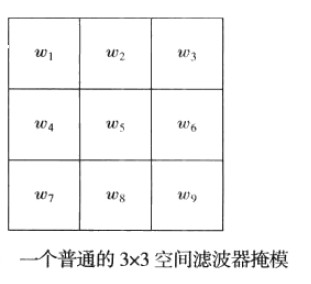## 2.1 - 孤立点的检测

$g(x,y)=\begin{cases} 1& | R(x,y)| \geq T\\ 0 & \text{ 其他 } \end{cases}$

## 2.2 - 线检测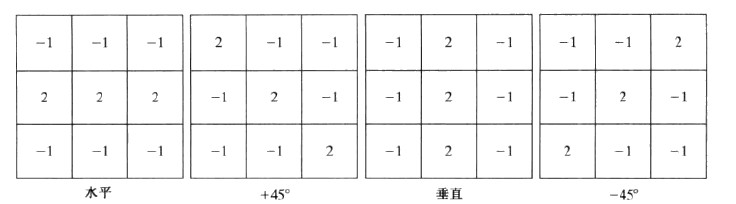## 2.4 边缘模型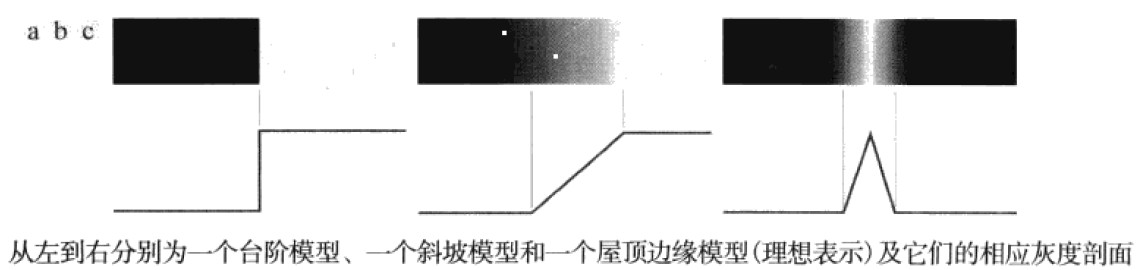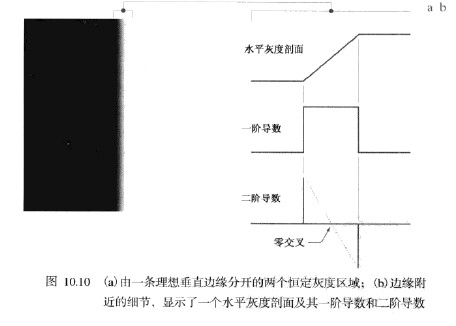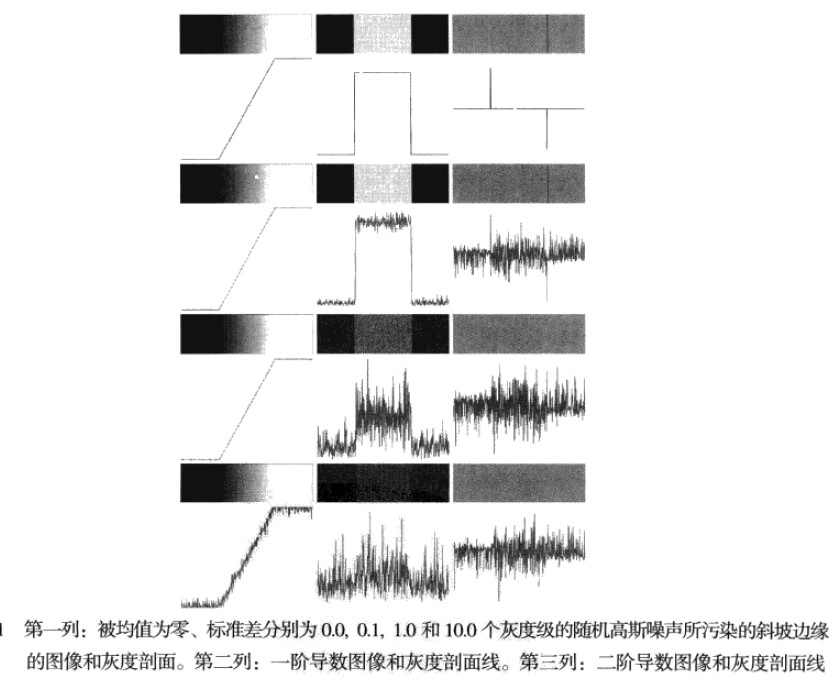1. 为降噪对图像进行平滑处理
2. 边缘点的检测
3. 边缘定位

### 2.4.1 - 基本的边缘检测算子

Roberts算子
Roberts算子以求对角像素之差为基础，该算子用于识别对角线方向的边缘：
$g_x=(z_9-z_5)和g_y=(z_8-z_6)$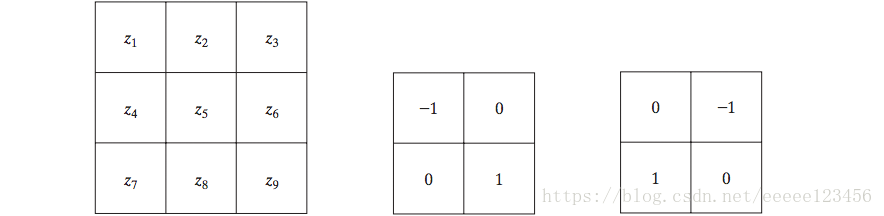Prewitt算子
Prewitt算子使用以$z_5$为中心的3x3领域对$g_x$$g_y$的近似如下式所示
$g_x=(z_7+z_8+z_9)-(z_1+z_2+z_3)$
$g_y=(z_3+z_6+z_9)-(z_1+z_4+z_7)$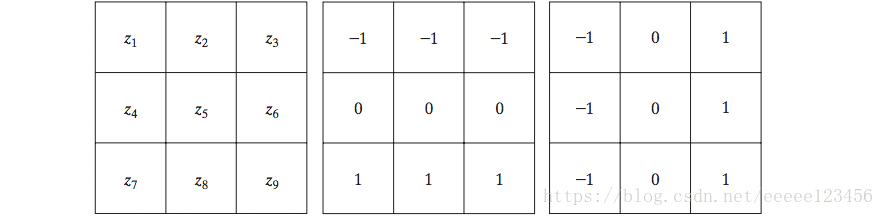Sobel算子
Sobel算子使用以$z_5$为中心的3x3领域对$g_x$$g_y$的近似如下式所示：
$g_x=(z_7+2z_8+z_9)-(z_1+2z_2+z_3)$
$g_y=(z_3+2z_6+z_9)-(z_1+2_z4+z_7)$

Sobel模板能较好地抑制（平滑）噪声地特性使得它更为可取，因为在处理导数时噪声抑制是一个重要地问题。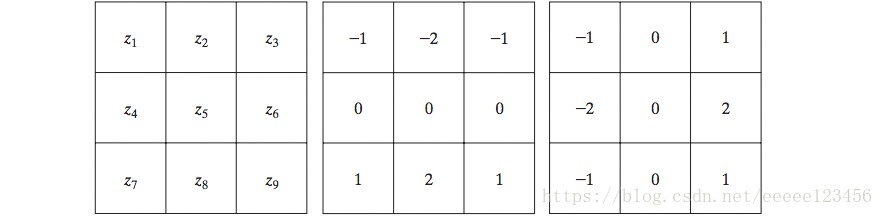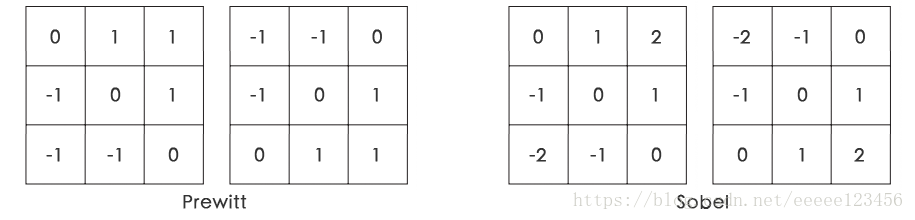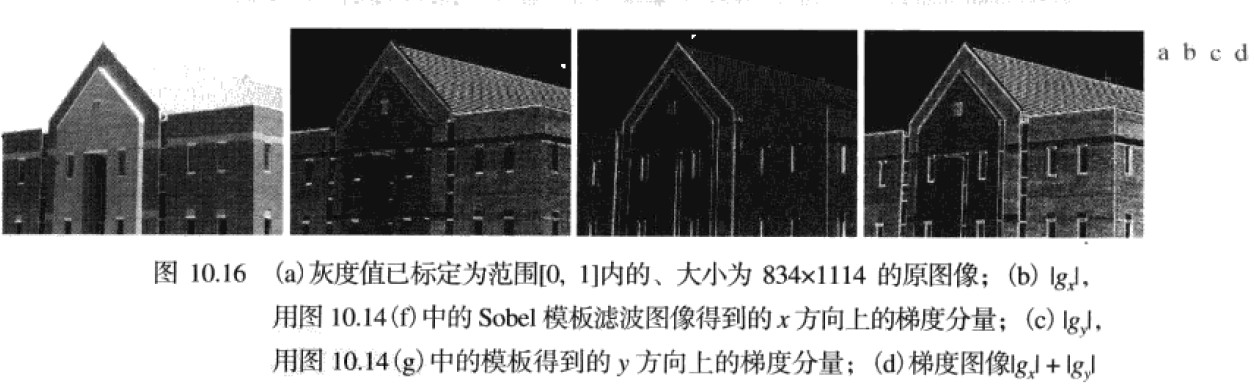# 3 - 成熟先进的边缘检测技术

## 3.1 - Marr-Hildreth边缘检测器

① 概念背景：

Marr和Hildreth证明了：

1. 灰度边缘与图像尺寸无关，因此他们的检测要求使用不同尺寸的算子；
2. 灰度的突然变化会在一阶导数中引起波峰或波谷，或在二阶导数中等效地引起零交叉

• 第一个和最重要的特点是它应该是一个能计算图像中每一点处的一阶导数或二阶导数的数字近似的微分算子。
• 第二个是它应能被“调整”以便在任何期望的尺寸上起作用
因此，大的算子也可以用于检测模糊边缘，小的算子也可以用于检测锐度集中的精细细节。

② 高斯拉普拉斯(LoG)：
Marr和Hildreth证明了：满足这些最令人满意的算子是滤波器$\triangledown^2G$,$\triangledown^2$是拉普拉斯算子（$\partial^2/\partial x^2+\partial^2/\partial y^2$）,而G是标准差为$\sigma$的二维高斯函数
$G(x,y)=e^{-\frac{x^2+y^2}{2\sigma^2}}$

$\triangledown^2G(x,y)=\frac{\partial^2G(x,y)}{\partial x^2}+\frac{\partial^2G(x,y)}{\partial y^2}=\frac{\partial}{\partial x}[\frac{-x}{\sigma^2}e^{-\frac{x^2+y^2}{2\sigma^2}}]+\frac{\partial}{\partial y}[\frac{-y}{\sigma^2}e^{-\frac{x^2+y^2}{2\sigma^2}}]$

$\triangledown^2G(x,y)=[\frac{x^2+y^2-2\sigma^2}{\sigma^4}]e^{-\frac{x^2+y^2}{2\sigma^2}}$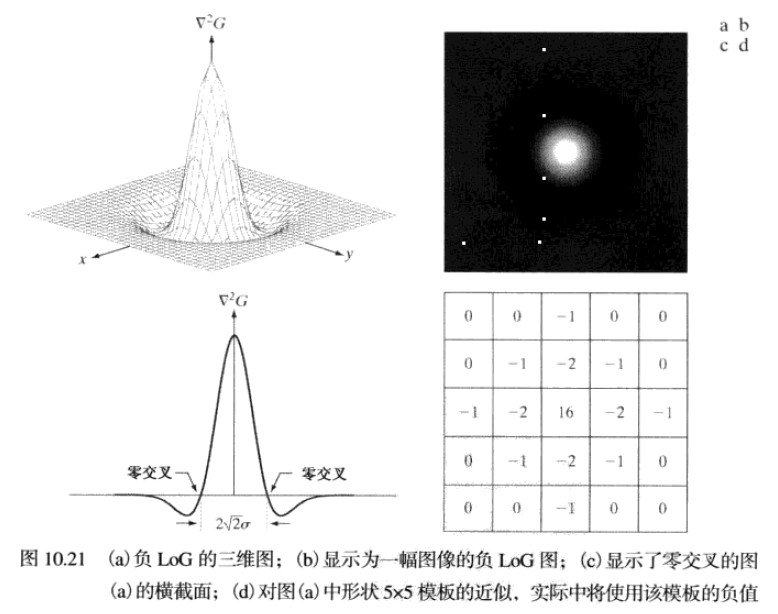③ Marr-Hildreth算法
Marr-Hildreth算法由LOG滤波器与一副输入图像f(x,y)卷积组成，即
$g(x,y)=[\triangledown^2G(x,y)]\bigstar f(x,y)$

KaTeX parse error: Expected 'EOF', got '\bigstarf' at position 30: …ledown^2[G(x,y)\̲b̲i̲g̲s̲t̲a̲r̲f̲(x,y)]

Marr-Hildreth算法小结

1. 用一个G(x,y)取样得到的nxn的高斯高斯低通滤波器对输入图像滤波。
2. 计算由第一步得到的图像的拉普拉斯
3. 找到步骤2所有图像的零交叉

import numpy as np
import matplotlib.pyplot as plt
import cv2

def edgesMarrHildreth(img, sigma):
"""
finds the edges using MarrHildreth edge detection method...
:param im : input image
:param sigma : sigma is the std-deviation and refers to the spread of gaussian
:return:
a binary edge image...
"""
size = int(2 * (np.ceil(3 * sigma)) + 1)

x, y = np.meshgrid(np.arange(-size / 2 + 1, size / 2 + 1), np.arange(-size / 2 + 1, size / 2 + 1))

normal = 1 / (2.0 * np.pi * sigma ** 2)

kernel = ((x ** 2 + y ** 2 - (2.0 * sigma ** 2)) / sigma ** 4) * np.exp(
-(x ** 2 + y ** 2) / (2.0 * sigma ** 2)) / normal  # LoG filter

kern_size = kernel.shape
log = np.zeros_like(img, dtype=float)

# applying filter
for i in range(img.shape - (kern_size - 1)):
for j in range(img.shape - (kern_size - 1)):
window = img[i:i + kern_size, j:j + kern_size] * kernel
log[i, j] = np.sum(window)

log = log.astype(np.int64, copy=False)

zero_crossing = np.zeros_like(log)

# computing zero crossing
for i in range(log.shape - (kern_size - 1)):
for j in range(log.shape - (kern_size - 1)):
if log[i][j] == 0:
if (log[i][j - 1] < 0 and log[i][j + 1] > 0) or (log[i][j - 1] < 0 and log[i][j + 1] < 0) or (
log[i - 1][j] < 0 and log[i + 1][j] > 0) or (log[i - 1][j] > 0 and log[i + 1][j] < 0):
zero_crossing[i][j] = 255
if log[i][j] < 0:
if (log[i][j - 1] > 0) or (log[i][j + 1] > 0) or (log[i - 1][j] > 0) or (log[i + 1][j] > 0):
zero_crossing[i][j] = 255

# plotting images
fig = plt.figure()
imgplot = plt.imshow(log, cmap='gray')
a.set_title('Laplacian of Gaussian')
imgplot = plt.imshow(zero_crossing, cmap='gray')
string = 'Zero Crossing sigma = '
string += (str(sigma))
a.set_title(string)
plt.show()

return zero_crossing

img = edgesMarrHildreth(img,4)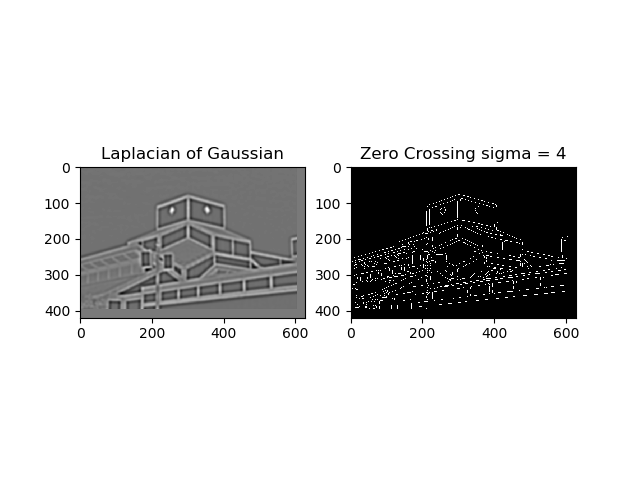（可以看到这个算法检测出了图像的边缘，但是有很多地方都是不连续的，那么之后我们会介绍如何检测边界和边缘连接）

## 3.2 - 坎尼边缘检测（Canny）

1. 低错误率。所有边缘都应被找到，并且应该没有伪相应，也就是检测到的边缘必须尽可能是真是的边缘
2. 边缘点应被很好的定位。已定位边缘必须尽可能接近真实边缘。也就是由检测器标记为边缘的点和真实边缘的中心之间的距离应该最小
3. 单一的边缘点响应。对于真实的边缘点，检测器仅应返回一个点。也就是真是边缘周围的局部最大数应该是最小的。这意味着在仅存一个单一边缘点到额位置，检测器不应指出多个边缘像素。

Canny工作的本质是，从数学上表达前面的三个准则，并试图找到这些表达式的最佳解，通常这是很困难的，但是我们可以使用高斯近似得出最优解：首先使用一个环形二维高斯函数平滑图像，计算结果的梯度，然后使用梯度幅度和方向来估计每一点的边缘强度与方向

$G(x,y)=e^{-\frac{x^2+y^2}{2\sigma^2}}$

$f_s(x,y)=G(x,y)\bigstar f(x,y)$

$M(x,y)=\sqrt {g_x^2+g_y^2}$
$\alpha(x,y)=arctan[\frac{g_y}{g_x}]$

1. 寻找最接近$\alpha(x,y)$的方向$d_k$
2. $M(x,y)$的值至少小于沿$d_k$的两个零邻居之一，零$g_N(x,y)=0$(抑制)；否则令$g_N(x,y) = M(x,y)$,得到最大非抑制后的图像$g_N(x,y)$

$g_{NH}=\left \{ g_N(x,y)\geq T_H\right\}$
$g_{NL}=\left \{ g_N(x,y)\geq T_L\right\}$
$g_{NH}= g_{NL}(x,y)-g_{NH}(x,y)$

$g_{NH}(x,y)$$g_{NL}(x,y)$的非零像素可分别视为“强”和“弱”边缘像素。其中$g_{NH}(x,y)$为边缘点，$g_{NL}(x,y)$为候选点，对于候选点，如果与边缘点邻近，就标记为边缘点。

1. $g_{NH}(x,y)$中定位一下个未被访问的边缘像素p
2. $g_{NL}(x,y)$中与p是8邻接的像素标记为有效边缘像素
3. $g_{NH}(x,y)$中的所有非零像素已被访问，则跳到步骤4，否走返回步骤1
4. $g_{NL}(x,y)$中未标记为有效边缘像素的所有像素置零
在这一过程的末尾，将来自$g_{NL}(x,y)$的所有非零像素附近到$g_{NH}(x,y)$，用Canny算子形成最终的输出图像。

Canny算法步骤

1. 用一个高斯滤波器平滑输入图像
2. 计算梯度幅度图像和角度图像
3. 对梯度幅度图像应用非最大抑制
4. 用双阈值处理和连接分析来检测并连接边缘（这相对Marr-Hildreth优化了边缘的连接使得检测的边缘更加完整）

OpenCV将这一算法封装成了一个函数

def Canny(image, threshold1, threshold2, edges=None, apertureSize=None, L2gradient=None): # real signature unknown; restored from __doc__
"""
Canny(image, threshold1, threshold2[, edges[, apertureSize[, L2gradient]]]) -> edges
.   @brief Finds edges in an image using the Canny algorithm @cite Canny86 .
.
.   The function finds edges in the input image and marks them in the output map edges using the
.   Canny algorithm. The smallest value between threshold1 and threshold2 is used for edge linking. The
.   largest value is used to find initial segments of strong edges. See
.   <http://en.wikipedia.org/wiki/Canny_edge_detector>
.
.   @param image 8-bit input image.
.   @param edges output edge map; single channels 8-bit image, which has the same size as image .
.   @param threshold1 first threshold for the hysteresis procedure.
.   @param threshold2 second threshold for the hysteresis procedure.
.   @param apertureSize aperture size for the Sobel operator.
.   @param L2gradient a flag, indicating whether a more accurate \f$L_2\f$ norm
.   \f$=\sqrt{(dI/dx)^2 + (dI/dy)^2}\f$ should be used to calculate the image gradient magnitude (
.   L2gradient=true ), or whether the default \f$L_1\f$ norm \f$=|dI/dx|+|dI/dy|\f$ is enough (


• 第一个参数是需要处理的原图像，该图像必须为单通道的灰度图；
• 第二个参数是阈值1；
• 第三个参数是阈值2。

Canny函数的使用很简单，只需指定最大和最小阈值即可。如下：

# coding=utf-8
import cv2
import numpy as np

cv2.imshow('img',img)
img = cv2.GaussianBlur(img, (3, 3), 0)
canny = cv2.Canny(img, 50, 150)

cv2.imshow('Canny', canny)
cv2.waitKey(0)
cv2.destroyAllWindows()
`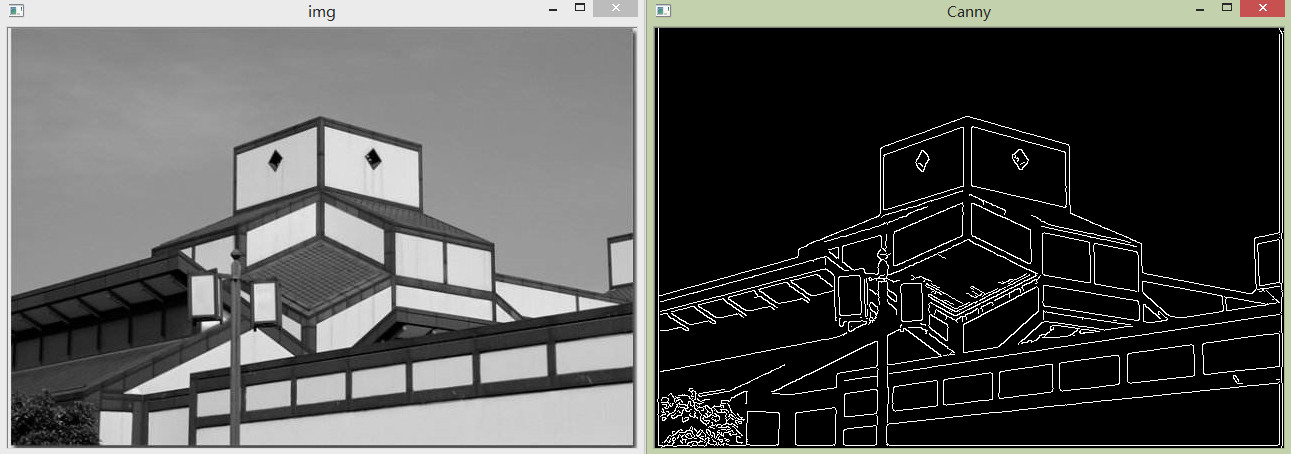## 【图像处理】RGB图像边缘检测

2018-10-03 15:46:39 u013921430 阅读数 5633
• ###### matlab边缘检测和图像分割

matlab边缘检测 <img src="https://img-bss.csdn.net/201903190616489027.jpg" alt="" /></p>

7课时 101分钟 981人学习 刘昱显
免费试看

## 灰度图边缘检测

在学习图像处理时，首先接触到的就是灰度图像的边缘检测，这是图像处理最基础的也是最重要的一环，熟悉图像边缘检测有助于我们学习其他的数字图像处理方法。由于图像的边缘区域会存在明显的像素值阶跃，因此边缘检测主要是通过获得图像灰度梯度，进而通过梯度大小和变化来判断图像边缘的。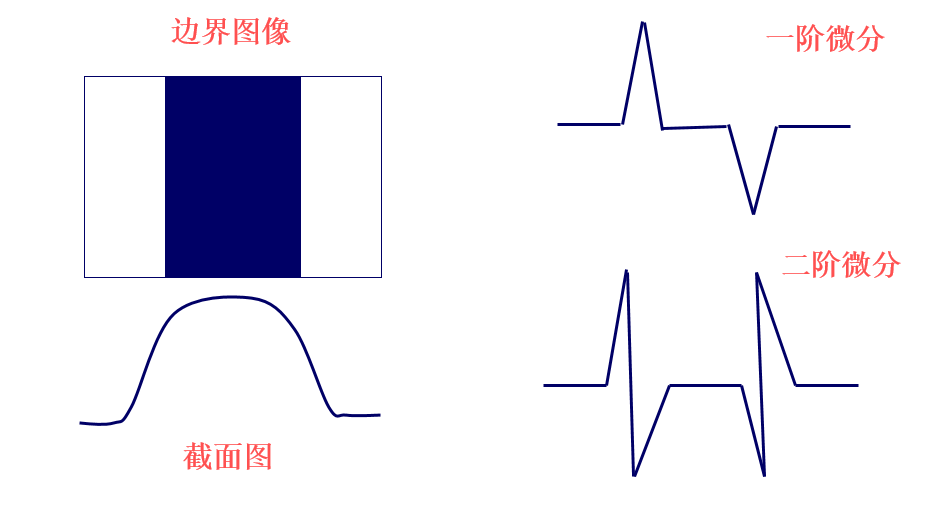因此，我们可以通过一阶差分；
$\Delta f_{x}(x,y)=f(x+1,y)-f(x,y)\\ \Delta f_{y}(x,y)=f(x,y+1)-f(x,y)$
或者二阶差分对边缘区域进行判断；
$\Delta f_{xx}(x,y)=f(x+1,y)+f(x-1,y)-2f(x,y)\\ \Delta f_{yy}(x,y)=f(x,y+1)+f(x,y-1)-2f(x,y)$
其中一阶差分可以判断边缘是否存在，二阶差分还可以根据正负号判断像素点在图像边缘亮的一侧还是暗的一侧。
其他的边缘检测方法还包括一些梯度算子，例如Prewitt算子、Sobel算子，Canny算子，LOG边缘检测算子等，在此不做说明。

## 彩色图边缘检测

RGB 图像使用三个通道存储像素信息，我们可以将这三个通道的信息看作是一个矢量，而矢量是不存在梯度的概念的，我们无法直接将上诉方法或算子直接用于RGB 图像，而且RGB图像单个通道的梯度信息又无法反映整体的梯度信息。
在《数字图像处理》（冈萨雷斯）中提到了一种针对彩色图像的边缘检测方法，这种方法由 Di Zenzo 等人在1986年提出，下面就一起看看这种方法如何得出。

在图像多通道图像$f(x,y)$ 中的某一点$P(x,y)$ 处，假设其梯度方向为$\theta$
$\Delta f=\left \| f(x+\varepsilon cos\theta,y+\varepsilon sin\theta) -f(x,y)\right \|$
为了便于计算，将计算绝对值换为计算平方，令
$\Delta f^{2}=\left \| f(x+\varepsilon cos\theta,y+\varepsilon sin\theta) -f(x,y)\right \|^{2}$
对$f(x+\varepsilon cos\theta,y+\varepsilon sin\theta)$进行二元泰勒展开；
\begin{aligned} f(x+\varepsilon cos\theta,y+\varepsilon sin\theta)&=f(x,y)+\sum_{i=1}^{m}(\varepsilon cos\theta\cdot\frac{\partial f_{i}(x,y)}{\partial x} +\varepsilon sin\theta\cdot\frac{\partial f_{i}(x,y)}{\partial y} )+o^{n}\\ &\approx f(x,y)+\sum_{i=1}^{m}(\varepsilon cos\theta\cdot\frac{\partial f_{i}(x,y)}{\partial x} +\varepsilon\cdot sin\theta\cdot\frac{\partial f_{i}(x,y)}{\partial y} ) \end{aligned}
其中$m$表示图像通道数目，为了方便表述使用$\frac{\partial f_{i}}{\partial x}$代替$\frac{\partial f_{i}(x,y)}{\partial x}$，而在求导时各个通道之间是相互独立的，则有；
$\Delta f^{2}\approx\sum_{i=1}^{m}(\varepsilon cos\theta\cdot\frac{\partial f_{i}}{\partial x} +\varepsilon sin\theta\cdot\frac{\partial f_{i}}{\partial y} )^{2}$
重新定义一个函数$G(\theta)$，令
\begin{aligned} G(\theta)&=\sum_{i=1}^{m}(\varepsilon cos\theta\cdot\frac{\partial f_{i}}{\partial x} +\varepsilon sin\theta\cdot\frac{\partial f_{i}}{\partial y} )^{2}\\ &=\varepsilon ^{2}(cos\theta^{2}\sum_{i=1}^{m}\left \|\frac{\partial f_{i}}{\partial x}\right \|^{2}+sin\theta^{2}\sum_{i=1}^{m}\left \|\frac{\partial f_{i}}{\partial y}\right \|^{2}+2sin\theta cos\theta \sum_{i=1}^{m}\frac{\partial f_{i}}{\partial x}\frac{\partial f_{i}}{\partial y}) \end{aligned}
进一步舍去式子中的$\varepsilon$ 项，令
$G(\theta)=cos\theta^{2}\sum_{i=1}^{m}\left \|\frac{\partial f_{i}}{\partial x}\right \|^{2}+sin\theta^{2}\sum_{i=1}^{m}\left \|\frac{\partial f_{i}}{\partial y}\right \|^{2}+2sin\theta cos\theta \sum_{i=1}^{m}\frac{\partial f_{i}}{\partial x}\frac{\partial f_{i}}{\partial y}$
为了进一步方便表述；令
$E=\sum_{i=1}^{m}\left \| \frac{\partial f_{i}}{\partial x} \right \|^{2}; F=\sum_{i=1}^{m}\left \|\frac{\partial f_{i}}{\partial y}\right \|^{2}; H=\sum_{i=1}^{m}\frac{\partial f_{i}}{\partial x}\frac{\partial f_{i}}{\partial y}$
$G(\theta)=cos\theta^{2}E+sin\theta^{2}F+2sin\theta cos\theta H$
现在$\theta$成为了式子中唯一的变量，再回到边缘的定义上，边缘的方向是图像像素梯度最大的方向。也就是说梯度的方向$\theta_{max}$ 会使$G(\theta)$ 取最大值，则；
$\theta_{max}=\underset{argmax}{G(\theta )}$
对$G(\theta)$ 进行求导；
$G(\theta )^{'}=-Esin2\theta +F sin2\theta+2 H cos2\theta$
令$G(\theta )^{'}=0$，得；
$tan ~2\theta_{max} =\frac{2H}{E-F}\\ \theta_{max}=\frac{1}{2}arctan(\frac{2H}{E-F}+k\pi)$
很明显$G(\theta )$ 是一个以$\pi$ 为周期的周期函数，如果只考虑区间$\left [ 0 ,\pi\right )$，且$\theta_{max}$ 落到该区间内，则会有另一个让$G(\theta )$取极值的解也落在该区域内，这个值是$\theta_{max}+ \frac{\pi}{2}$或者$\theta_{max}-\frac{\pi}{2}$。但是不论如何这两个解有一个让$G(\theta )$取极大值，另一个让其取极小值，两个角度相差 90°。

说到这里大家应该都明白了，两个角度对应的方向是相互垂直的，一个是垂直于边缘的方向，也就是边缘的法向，此时梯度取最大值。另一个是平行于边缘的方向，是边缘的切向，此时梯度取极小值。

### RGB图像的边缘检测

在RGB图像中，$m=3$，再令；
$\overset{\rightarrow }{u}=\frac{\partial R}{\partial x}\overset{\rightarrow }{r}+\frac{\partial G}{\partial x}\overset{\rightarrow }{g}+\frac{\partial B}{\partial x}\overset{\rightarrow }{b}$
$\overset{\rightarrow }{v}=\frac{\partial R}{\partial y}\overset{\rightarrow }{r}+\frac{\partial G}{\partial y}\overset{\rightarrow }{g}+\frac{\partial B}{\partial y}\overset{\rightarrow }{b}$
其中$\overset{\rightarrow }{r}$$\overset{\rightarrow }{g}$$\overset{\rightarrow }{b}$分别代表不同颜色分量的单位向量，则
$g_{xx}=E=\overset{\rightarrow }{u}^{\tiny{T}}\overset{\rightarrow }{u}=\left \| \frac{\partial R}{\partial x} \right \|^{2}+\left \| \frac{\partial G}{\partial x} \right \|^{2}+\left \| \frac{\partial B}{\partial x} \right \|^{2}$
$g_{yy}=F=\overset{\rightarrow }{v}^{\tiny{T}}\overset{\rightarrow }{v}=\left \| \frac{\partial R}{\partial y} \right \|^{2}+\left \| \frac{\partial G}{\partial y} \right \|^{2}+\left \| \frac{\partial B}{\partial y} \right \|^{2}$
$g_{xy}=H=\overset{\rightarrow }{u}^{\tiny{T}}\overset{\rightarrow }{v}=\frac{\partial R}{\partial x}\frac{\partial R}{\partial y}+\frac{\partial G}{\partial x}\frac{\partial G}{\partial y}+\frac{\partial B}{\partial x}\frac{\partial B}{\partial y}$
在利用Di Zenzo 提出的方法求得$\theta_{max}$ 后，将以上符号带入到$G(\theta)$，可以计算出像素点梯度大小为
$G(\theta)=\left \{ \frac{1}{2}\left [ (g_{xx}+g_{yy}) +(g_{xx}-g_{yy})cos~2\theta_{max} +2g_{xy}sin~2\theta_{max} \right ]\right \}^{\frac{1}{2}}$
进而可以根据梯度大小进行边缘检测。

## 参考资料

1. S. Di Zenzo, A note on the gradient of a multi-image, Computer Vision, Graphics, and Image Processing 33 (1) (1986) 116–125.
2. 《数字图像处理》 (冈萨雷斯)